## Excel SUMIFS function

The SUMIFS function in Excel can help to sum cell values based on multiple criteria, this tutorial will explain the SUMIFS function's syntax and some examples for using this function.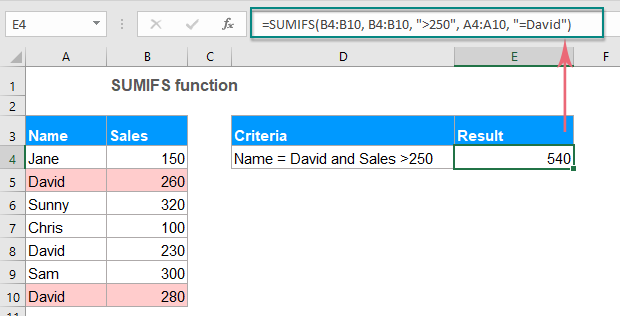#### Syntax:

The syntax for the SUMIFS function in Excel is:

=SUMIFS(sum_range, criteria_range1, criteria1, [criteria_range2, criteria2], ...)

#### Arguments:

• sum_range: Required. The cells or range of cells that you want to sum. Only cells with numbers are summed, blank cells and text values are ignored.
• criteria_range1: Required. The first range that you want to apply the criteria1 against.
• criteria1: Required. The first condition to be used on range1.
• criteria_range2, criteria2, … : Optional. Other additional ranges and their associated criteria.

Notes:

• 1. The SUMIFS function can handle up to 127 pairs of criteria_range and criteria arguments.
• 2. The criteria_range argument must contain the same number of rows and columns as the sum_range argument.
• 3. The type of the criteria can be: number, logical expression, cell reference, text, date or other Excel function, if the criteria is a text string or an expression, it must be enclosed in double-quotes.
• 4. The SUMIFS function is case-insensitive. For example, the text strings "excel" and "EXCEL" will be considered to be equal.
• 5. The SUMIFS function works with AND logic, it means that a cell in the sum range is summed only if it meets all of the specified criteria.

#### Return:

Return the summation for a list of numbers that meet all specific criteria.

#### Example 1: Basic use of SUMIFS function

For example, I have a table with Product, Name, Sales and Price columns as below screenshot shown, now, I want to find out the sum of total price which product is KTE and the sales is greater than 200.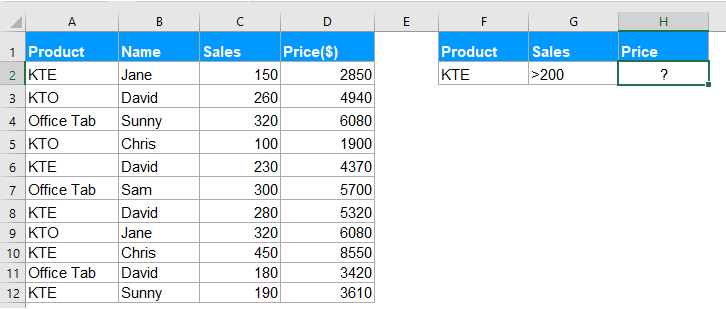Please apply the following formula into a blank cell:

=SUMIFS(D2:D12, A2:A12, "KTE", C2:C12, ">200")
• Tips: In the above formula:
• D2:D12: is the range of cells you want to sum.
• A2:A12, "KTE": The first criteria_range and criteria that you want to sum values based on.
• C2:C12, ">200": The second criteria_range and criteria that you want to sum values based on.

And then, press Enter key to get the result you need. See screenshot: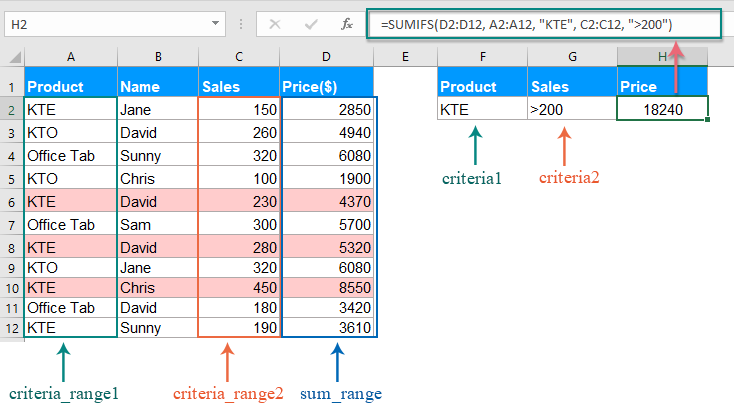Note: You can also use the cell reference to replace the condition in the above formula as this: =SUMIFS(D2:D12, A2:A12,F2, C2:C12, G2).

#### Example 2: SUMIFS function with dates in Excel

When you have a list of dates, and you need to sum the cell values between two given dates, the SUMIFS function can solve this task quickly and easily.

Please use the below formula into a blank cell:

=SUMIFS(C2:C12, A2:A12, ">=4/1/2019", A2:A12, "<=5/31/2019")
• Tips: In the above formula:
• C2:C12: is the range of cells you want to sum.
• A2:A12, ">=4/1/2019": The first criteria_range and criteria that you want to sum values based on.
• A2:A12, "<=5/31/2019": The second criteria_range and criteria that you want to sum values based on.

And then, press Enter key, the total price of between the two given dates has been calculated, see screenshot: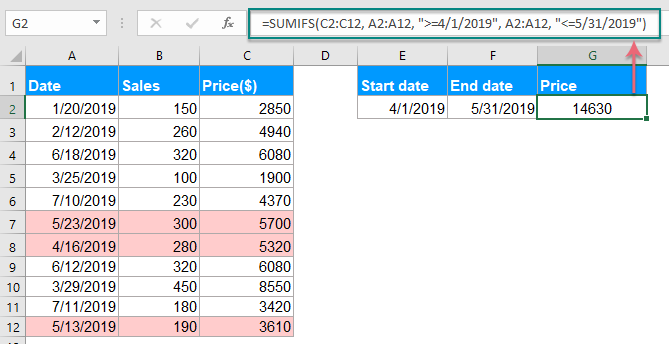Note: You can also use the cell reference to replace the condition in the above formula as this: =SUMIFS(C2:C12, A2:A12, ">=" &E2, A2:A12, "<=" &F2).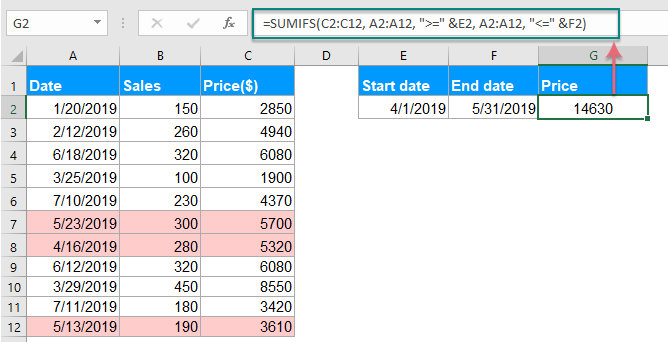#### Example 3: SUMIFS function with blank and non-blank cells

Supposing, you have a range of data as following screenshot shown, now, if you want to sum the total price when the cells in Column B are not blank cell and the cells in Column C are blank cell, how could you do?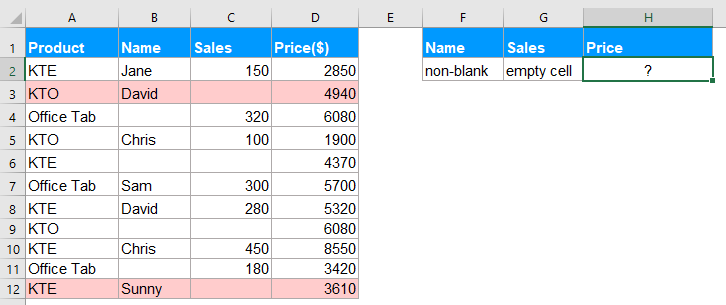To solve this task, you should use the following formula:

=SUMIFS(D2:D12, B2:B12,"<>", C2:C12,"=")

Then, press Enter key to get the result as you need. See screenshot: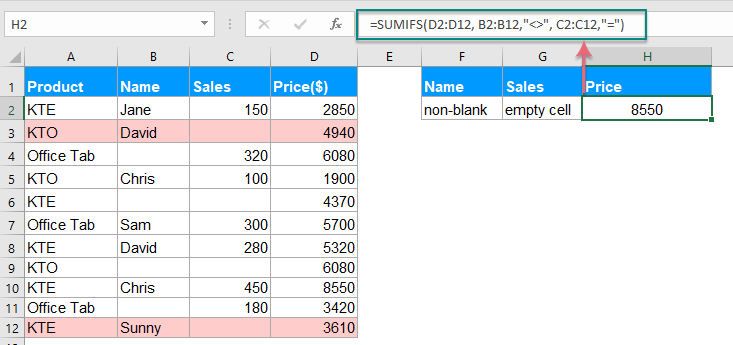#### Example 4: SUMIFS function with multiple criteria and OR logic

By default, the SUMIFS function only allows AND logic, but sometimes, you may need to sum cell values based on multiple criteria with OR logic in Excel.

For example, if you have a range of data as below screenshot shown, to sum the total price from David, Jane or Sunny which sales is greater than 200.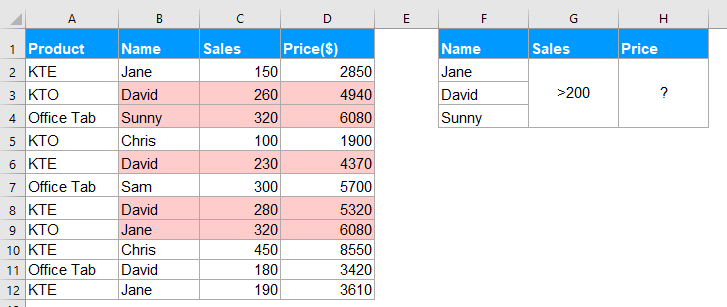Formula1: SUMIFS() + SUMIFS() + SUMIFS() +…

To solve this job, you can combine the SUMIFS function together for summing cells with OR logic. Please apply the below formula:

=SUMIFS(D2:D12, B2:B12, "Jane", C2:C12, ">200")+SUMIFS(D2:D12, B2:B12, "David", C2:C12, ">200")+SUMIFS(D2:D12, B2:B12, "Sunny", C2:C12, ">200")

And then, press Enter key to get the correct result as you need: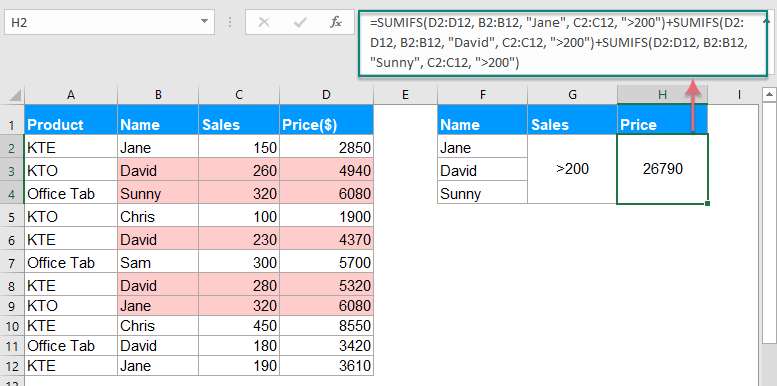Formula2: SUM(SUMIFS({}))

When there are multiple OR logic, the above formula will be somewhat difficult, to simplify the above formula, you can create an array formula with SUM and SUMIFS functions.

Please enter or copy the following formula into a blank cell:

=SUM(SUMIFS(D2:D12,B2:B12,{"Jane","David","Sunny"}, C2:C12, ">200"))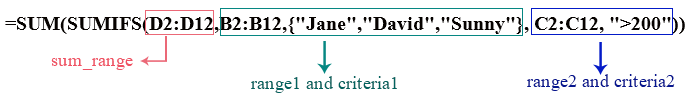Then, press Ctrl + Shift + Enter keys together to get the result as below screenshot shown: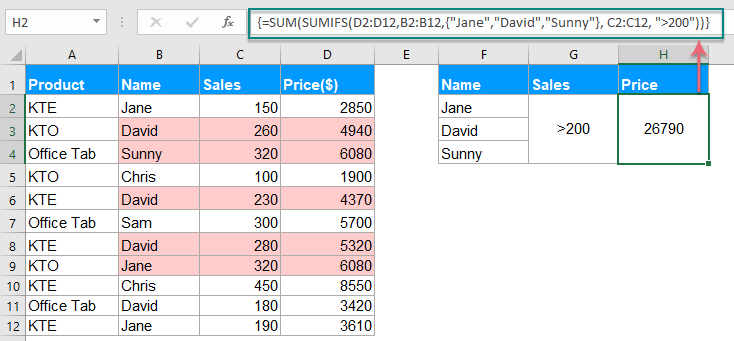### The Best Office Productivity Tools

#### Kutools for Excel - Helps You To Stand Out From Crowd

 Popular Features: Find, Highlight or Identify Duplicates  |  Delete Blank Rows  |  Combine Columns or Cells without Losing Data  |  Round without Formula ... Super VLookup: Multiple Criteria  |  Multiple Value  |  Across Multi-Sheets  |  Fuzzy Lookup... Adv. Drop-down List: Easy Drop Down List  |  Dependent Drop Down List  |  Multi-select Drop Down List... Column Manager: Add a Specific Number of Columns  |  Move Columns  |  Toggle Visibility Status of Hidden Columns  |  Compare Columns to Select Same & Different Cells ... Featured Features: Grid Focus  |  Design View  |  Big Formula Bar  |  Workbook & Sheet Manager | Resource Library (Auto Text)  |  Date Picker  |  Combine Worksheets  |  Encrypt/Decrypt Cells  |  Send Emails by List  |  Super Filter  |  Special Filter (filter bold/italic/strikethrough...) ... Top 15 Toolsets:  12 Text Tools (Add Text, Remove Characters ...)  |  50+ Chart Types (Gantt Chart ...)  |  40+ Practical Formulas (Calculate age based on birthday ...)  |  19 Insertion Tools (Insert QR Code, Insert Picture from Path ...)  |  12 Conversion Tools (Numbers to Words, Currency Conversion ...)  |  7 Merge & Split Tools (Advanced Combine Rows, Split Excel Cells ...)  |  ... and more

Kutools for Excel Boasts Over 300 Features, Ensuring That What You Need is Just A Click Away...#### Office Tab - Enable Tabbed Reading and Editing in Microsoft Office (include Excel)

• One second to switch between dozens of open documents!
• Reduce hundreds of mouse clicks for you every day, say goodbye to mouse hand.
• Increases your productivity by 50% when viewing and editing multiple documents.
• Brings Efficient Tabs to Office (include Excel), Just Like Chrome, Edge and Firefox.